## Octahedron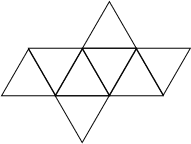A Platonic Solid () with six Vertices, 12 Edges, and eight equivalent Equilateral Triangular faces (), given by the Schläfli Symbol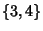. It is also Uniform Polyhedronwith the Wythoff Symbol. Its Dual Polyhedron is the Cube. Like the Cube, it has theOctahedral Group of symmetries. The octahedron can be Stellated to give the Stella Octangula.

The solid bounded by the two Tetrahedra of the Stella Octangula (left figure) is an octahedron (right figure; Ball and Coxeter 1987).

In one orientation (left figure), the Vertices are given by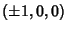,,. In another orientation (right figure), the vertices are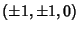and. In the latter, the constituent Triangles are specified by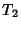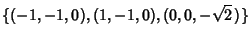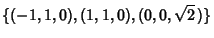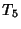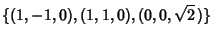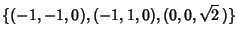The face planes are, so a solid octahedron is given by the equation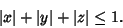(1)

A plane Perpendicular to a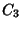axis of an octahedron cuts the solid in a regular Hexagonal Cross-Section (Holden 1991, pp. 22-23). Since there are four such axes, there are four possibly Hexagonal Cross-Sections. Faceted forms are the Cuboctatruncated Cuboctahedron and Tetrahemihexahedron.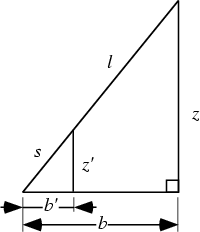Let an octahedron be lengthon a side. The height of the top Vertex from the square plane is also the Circumradius(2)

where(3)

is the diagonal length, so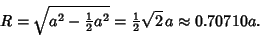(4)(5)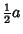(6)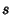(7)

so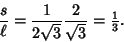(8)

Now use similar Triangles to obtain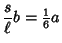(9)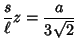(10)(11)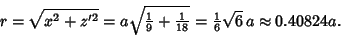(12)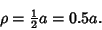(13)

The Area of one face is the Area of an Equilateral Triangle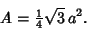(14)

The volume is two times the volume of a square-base pyramid,(15)

The Dihedral Angle is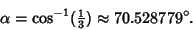(16)

See also Octahedral Graph, Octahedral Group, Octahedron 5-Compound, Stella Octangula, Truncated Octahedron

References

Davie, T. The Octahedron.'' http://www.dcs.st-and.ac.uk/~ad/mathrecs/polyhedra/octahedron.html.

Holden, A. Shapes, Space, and Symmetry. New York: Dover, 1991.